Home » 7 Steps of Data Exploration & Preparation – Part 1

# 7 Steps of Data Exploration & Preparation – Part 1

### Introduction

I have been a Business Analytics professional for close to three years now. In my initial days, one of my mentor suggested me to spend ample time on hypothesis generation before touching the data. Following his advice has served me well.

Hypothesis generation requires you to have structured thinking whereas data exploration requires patience to slice and dice data in multiple ways. In this article, I will focus on the steps required to clean and understand data in a comprehensive way.

To improve your structured thinking , I would suggest you to check out the flawless post written by Kunal – “Tools to Improve structure Thinking“.### Steps of Data Exploration and Preparation

Remember the quality of your inputs decide the quality of your output. So once you have your business hypothesis ready, it makes sense to spend a lot of time and effort here. By my personal estimate, data exploration, cleaning and preparation would take up to 70% of your total project time.

Below are the steps involved to understand, clean and prepare your data for building your predictive model:

1. Variable Identification
2. Univariate Analysis
3. Bi-variate Analysis
4. Missing values treatment
5. Outlier treatment
6. Variable transformation
7. Variable creation

Finally, we will need to iterate over steps 4 – 7 multiple times before we come up with our refined model.

Let’s now study each stage in  detail:-

### Step-1: Variable Identification

First, identify Predictor (Input) and Target (output) variables. Next, identify the data type and category of the variables.

Let’s understand this step more clearly by taking an example.

Example:- Suppose, we want to predict, whether the students will play cricket or not (refer below data set). Here you need to identify predictor variables, target variable, data type of variables and category of variables.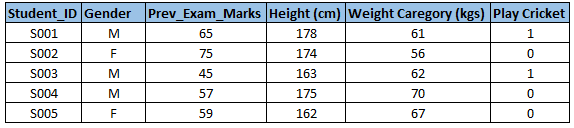Below, the variables have been defined in different category: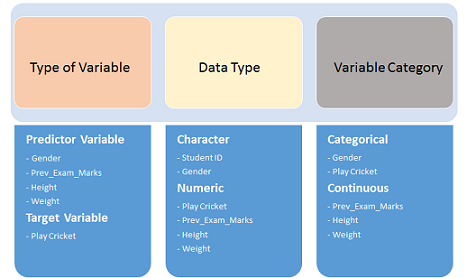### Step-2: Univariate Analysis

At this stage, we explore variables one by one. Method to perform uni-variate analysis will depend on whether the variable type is categorical or continuous. Let’s look at these methods and statistical measures for categorical and continuous variables individually:

Continuous Variables:- In case of continuous variables, we need to understand the central tendency and spread of the variable. These are measured using various statistical metrics visualization methods as shown below: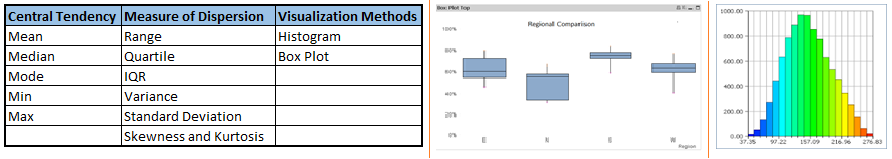Note: Univariate analysis is also used to highlight missing and outlier values. In the upcoming part of this series, we will look at methods to handle missing and outlier values. To know more about these methods, you can refer course descriptive statistics from Udacity.

Categorical Variables:- For categorical variables, we’ll use frequency table to understand distribution of each category. We can also read as percentage of values under each category. It can be be measured using two metrics, Count and Count% against each category. Bar chart can be used as visualization.

### Stage-3: Bi-variate Analysis

Bi-variate Analysis finds out the relationship between two variables. Here, we look for association and disassociation between variables at a pre-defined significance level. We can perform bi-variate analysis for any combination of categorical and continuous variables. The combination can be: Categorical & Categorical, Categorical & Continuous and Continuous & Continuous. Different methods are used to tackle these combinations during analysis process.

Let’s understand the possible combinations in detail:

Continuous & Continuous: While doing bi-variate analysis between two continuous variables, we should look at scatter plot. It is a nifty way to find out the relationship between two variables. The pattern of scatter plot indicates the relationship between variables. The relationship can be linear or non-linear.Scatter plot shows the relationship between two variable but does not indicates the strength of relationship amongst them. To find the strength of the relationship, we use Correlation. Correlation varies between -1 and +1.

• -1: perfect negative linear correlation
• +1:perfect positive linear correlation and
• 0: No correlation

Correlation can be derived using following formula:

Correlation = Covariance(X,Y) / SQRT( Var(X)* Var(Y))

Various tools have function or functionality to identify correlation between variables. In Excel, function CORREL() is used to return the correlation between two variables and SAS uses procedure PROC CORR to identify the correlation. These function returns Pearson Correlation value to identify the relationship between two variables: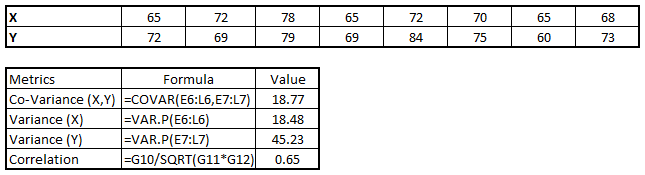In above example, we have good positive relationship(0.65) between two variables X and Y.

Categorical & Categorical: To find the relationship between two categorical variables, we can use following methods:

• Two-way table: We can start analyzing the relationship by creating a two-way table of count and count%. The rows represents the category of one variable and the columns represent the categories of the other variable. We show count or count% of observations available in each combination of row and column categories.
• Stacked Column Chart: This method is more of a visual form of Two-way table.• Chi-Square Test: This test is used to derive the statistical significance of relationship between the variables. Also, it tests whether the evidence in the sample is strong enough to generalize that the relationship for a larger population as well. Chi-square is based on the difference between the expected and observed frequencies in one or more categories in the two-way table. It returns probability for the computed chi-square distribution with the degree of freedom.

Probability of 0: It indicates that both categorical variable are dependent

Probability of 1: It shows that both variables are independent.

Probability less than 0.05: It indicates that the relationship between the variables is significant at 95% confidence. The chi-square test statistic for a test of independence of two categorical variables is found by: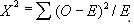where O represents the observed frequency. E is the expected frequency under the null hypothesis and computed by: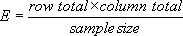From previous two-way table, the expected count for product category 1 to be of small size is  0.22. It is derived by taking the row total for Size (9) times the column total for Product category (2) then dividing by the sample size (81). This is procedure is conducted for each cell. Statistical Measures used to analyze the power of relationship are:

• Cramer’s V for Nominal Categorical Variable
• Mantel-Haenszed Chi-Square for ordinal categorical variable.

Different data science language and tools have specific methods to perform chi-square test. In SAS, we can use Chisq as an option with Proc freq to perform this test.

Categorical & Continuous: While exploring relation between categorical and continuous variables, we can draw box plots for each level of categorical variables. If levels are small in number, it will not show the statistical significance. To look at the statistical significance we can perform Z-test, T-test or ANOVA.

Example: Suppose, we want to test the effect of five different exercises. For this, we recruit 20 men and assign one type of exercise to 4 men (5 groups). Their weights are recorded after a few weeks. We need to find out whether the effect of these exercises on them is significantly different or not. This can be done by comparing the weights of the 5 groups of 4 men each.

### End Notes:

In this article, we have looked at the first three stages of Data Exploration, Variable Identification, Uni-Variate and Bi-Variate analysis. We have also looked at various statistical and visual methods to identify the relationship between variables. In the next article we will look at the methods to deal with missing and outlier values in detail. Stay Tuned !

### If you like what you just read & want to continue your analytics learning, subscribe to our emails, follow us on twitter or like our facebook page.

You can also read this article on our Mobile APP•Diptesh says:

Simply superb…

•Jasmeet says:

A good informative post again. Somehwere in the Step 3, you mentioned , ” GOOD positive relationship(0.65) between two variables X and Y.” Is it really a good practice to call positive correlations as GOOD or BAD. How about calling them, Strong Positive Correlation or Weak Positive Correlation!

•K Sridharan says:

Well explained; looking forward to next installement

•Gaurav says:

Nice Article!

•Hassine Saidane says:

I believe that some commercial data mining/stat software have tools to do EDA, Data Cleaning , Preparation , Transformation, Variable selection, and even model selection. Do you know which ones and how they do it (algorithms). especially for transformation and variable selection?

Thanks

•Sunny says:

Crisp and sharp deck!

•Raj says:

Very good article. Where is the part 2, by the way?

•akash9129 says:

Hi Sunil,

Is this the base for learning how to build models while analysing data? Be it R, SAS or excel ?

•Charllie says:

So if we found the two variables are linear, can we just neglect one of them?

•Sunil M says:

Hi Sunil Ray, Thanks for the wonderful post.

I just wanted one clarification…

Regarding your explanation on performing bivariate analysis between continuous and discrete variable, can you elaborate how Z or T or ANOVA can be used ?
Does these tests explain the association or disassociation between continuous and discrete variable. ?